• Call Now

1800-102-2727•

# Lines: Definition, Types, General Equation of Line, Practice Problems, FAQ’s

You may have noticed various objects in your surroundings such as the edge of a table, pen, edge of a notebook and many more objects. They have something in common that is all of them are lines. A line is a one-dimensional shape with zero thickness but length can extend in both directions. Basically, it is a combination of various points which are moving in opposite directions and when these points come too close together they form a line. So, let’s understand this interesting topic in this article.

## Line: Definition

A group of points which are moving in a straight path but in opposite directions forms a Line. It has no breadth, no depth (curvature) and no termination means ‘it will not end’ so it keeps moving in a straight path endlessly in opposite directions.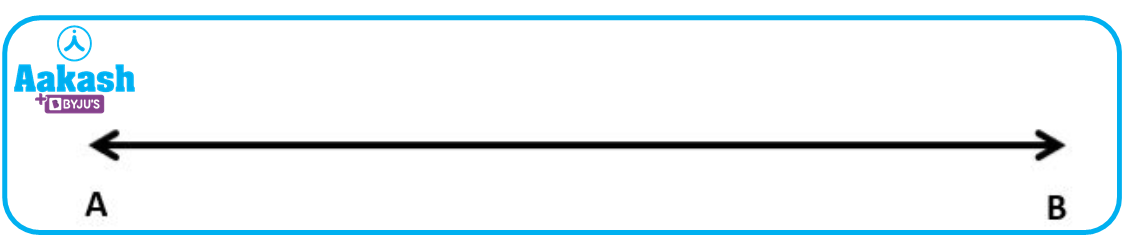### Line Segment

A line is said to be a line segment when it has two fixed endpoints. Line segment is a part of line. The length of the line segment is always constant, which is the distance between the two endpoints. Below shown is a line segment XY.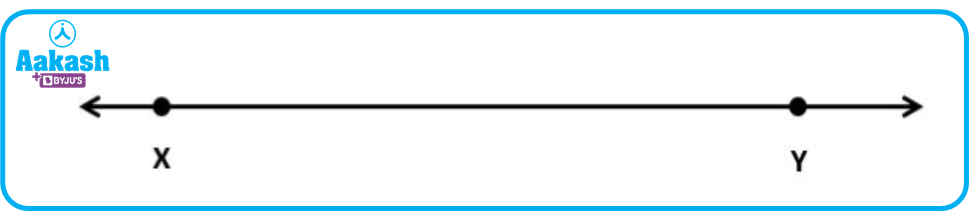### Ray

A line is said to be ray when it has a fixed starting point but no endpoint. It can extend infinitely in one direction. Since a ray has no endpoint so we cannot measure its length.

For example,

Sunray is an example of a ray. The Sun is the starting point or point of origin and its ray extends indefinitely.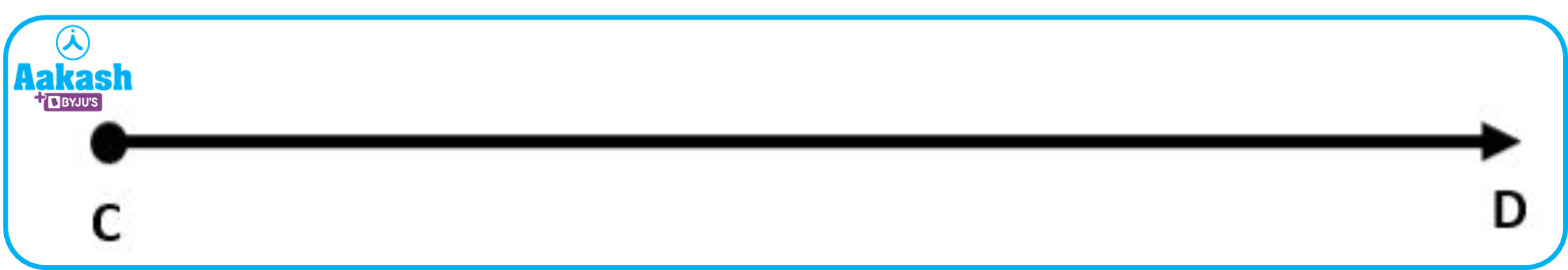## Line: Types

The different types of lines are:

1. Horizontal Line
2. Vertical Line
3. Parallel Lines
4. Perpendicular Lines
5. Intersecting Lines

They are the part of geometry or we can say that they are the foundation of geometry.

Now, let’s understand each one of them in brief

 Type of Line Definition Diagram Horizontal Line A line which moves in a straight path from left to right and vice-versa or a line which is parallel to the X- axis is a Horizontal Line.Vertical Line A line is said to be vertical when it is perpendicular to the X- axis.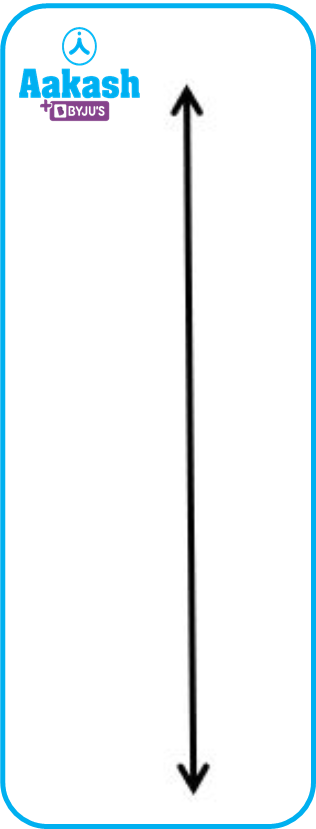Parallel Lines Two lines are said to be parallel when no two lines coincide or meet each other at any point.The distance between parallel lines is constant everywhere.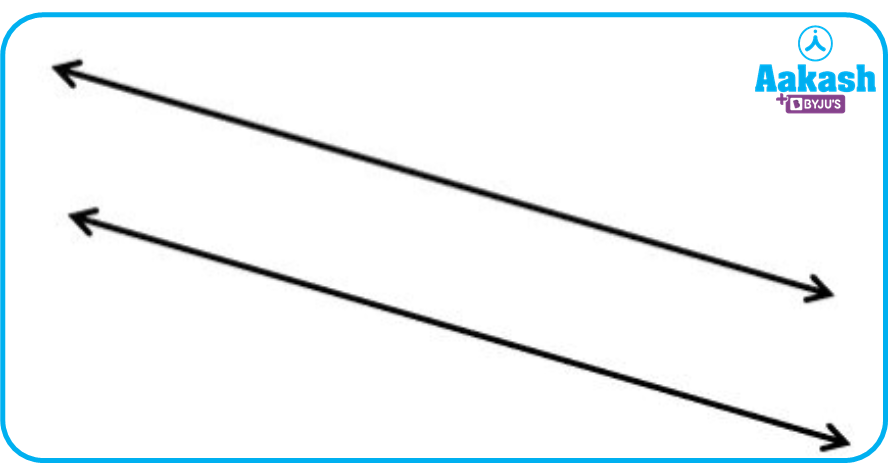Perpendicular Lines When two lines intersect each other and form an angle of 900 or make a right angle, then they are said to be perpendicular Lines.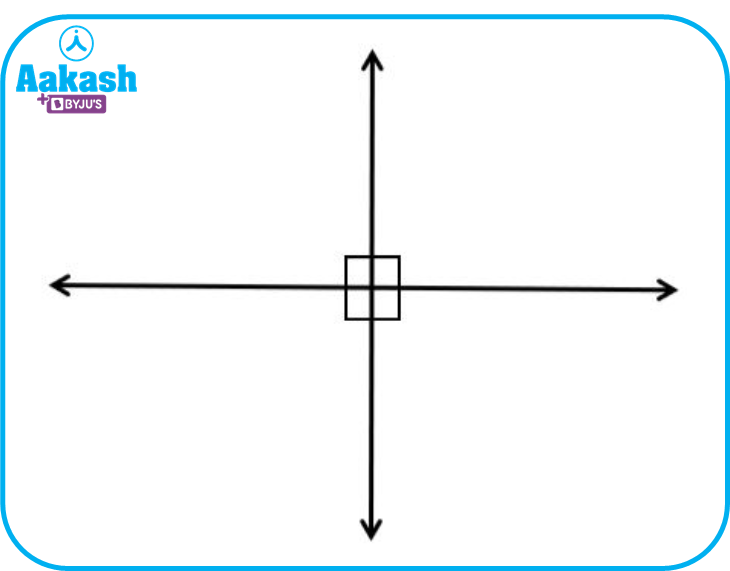Intersecting Lines When two lines meet at any point or cross each other at any point then they are said to be interesting lines.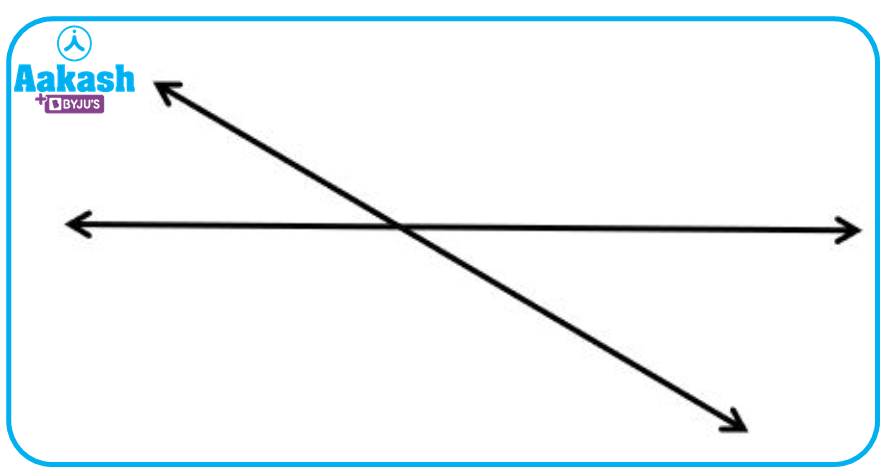## General Equation of Line

We represent the general equation of a line in two variables of one degree as

Ax+By+C=0

Where A and B should not be zero simultaneously; and A, B, and C are the real numbers.

The general equation of a line is also known as a linear equation in two variables.

For example:

2x+6y-3=0, where A=2, B=6 and C=-3

## Various Forms of Equation of Line

1. Point-slope form:

In this form, the line is passing through a point (x0,y0) and the slope is m then the equation of the line is given by:1. Two-point form:

In this form, the line passes through two points say (x1,y1) and (x2,y2) then the equation of line will be given as1. Slope-intercept form:

In this form, a line with slope m cuts the y-axis at a distance c from the origin. The distance is called the Y-intercept of the line. The line always passes through the point(0,c), therefore by slope-intercept form, the equation of the line will be given as1. Intercept form:

In this form, the line makes x-intercepts a and y-intercepts b on axes and meets the x-axis at (a,0) and the y-axis at (0,b). Now, by two-point form i.e.,By putting the value, we getBy rearranging the equation, we getThis is the intercept-form.

## Practice Problems

1. #### Find the equation of the line for x-axis and y-axis.

Solution: The equation for the x-axis is y=0 and for the y-axis is x=0.

1. #### Write the equation of the line with slope 5 and passing through (2,1).

Solution: We have value of the slope i.e., m=5 and (x0,y0) = (2,1)

Now, by using1. #### Write the equation of a line when passing through (1,2) and (-4,6).

Solution: Here, the line is passing through (1,2) and (-4,6)

Now,1. #### Find the y-intercept of a line 2x+5y=10.

Solution: The given equation is 2x+y=10

Now,Hence, the y-intercept is 2.

1. #### Find the equation of a line passing through (1,4) and m=2.

Solution: We have value of the slope i.e., m=2 and (x0,y0) = (1,4)

Now, by using1. #### What is a line?

A line is a one-dimensional figure with no endpoints.

1. #### What is the relation between the slope of two parallel lines?

The slope of two parallel lines is equal.

1. #### When is the slope of a line zero?

The slope of a line is zero only when the line is parallel to the x-axis or the line itself is the x-axis.

1. #### Do lines have ends?

No, Lines do not have endpoints. Although line segment has 2 end points and ray has 1 end point.Talk to our expert
Resend OTP Timer =
By submitting up, I agree to receive all the Whatsapp communication on my registered number and Aakash terms and conditions and privacy policy